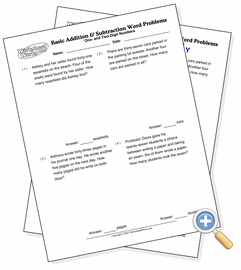# One- and Two-Digit Numbers

## Basic Addition & Subtraction Word ProblemsSolve word problems involving addition and subtraction with one- and two-digit numbers

This collection combines word problems involving addition with one- and two-digit addends and subtraction from less than 100 with one-digit subtrahends.